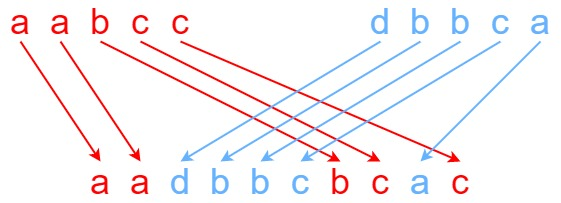# GeetCode Hub

Given strings `s1`, `s2`, and `s3`, find whether `s3` is formed by an interleaving of `s1` and `s2`.

An interleaving of two strings `s` and `t` is a configuration where they are divided into non-empty substrings such that:

• `s = s1 + s2 + ... + sn`
• `t = t1 + t2 + ... + tm`
• `|n - m| <= 1`
• The interleaving is `s1 + t1 + s2 + t2 + s3 + t3 + ...` or `t1 + s1 + t2 + s2 + t3 + s3 + ...`

Note: `a + b` is the concatenation of strings `a` and `b`.

Example 1:```Input: s1 = "aabcc", s2 = "dbbca", s3 = "aadbbcbcac"
Output: true
```

Example 2:

```Input: s1 = "aabcc", s2 = "dbbca", s3 = "aadbbbaccc"
Output: false
```

Example 3:

```Input: s1 = "", s2 = "", s3 = ""
Output: true
```

Constraints:

• `0 <= s1.length, s2.length <= 100`
• `0 <= s3.length <= 200`
• `s1`, `s2`, and `s3` consist of lowercase English letters.

Follow up: Could you solve it using only `O(s2.length)` additional memory space?

class Solution { public boolean isInterleave(String s1, String s2, String s3) { } }